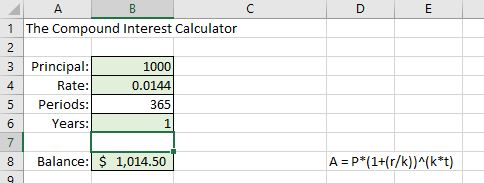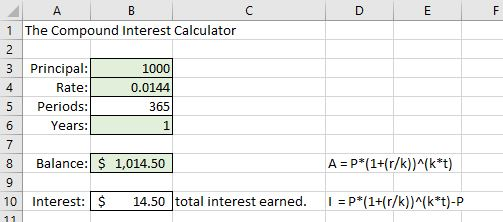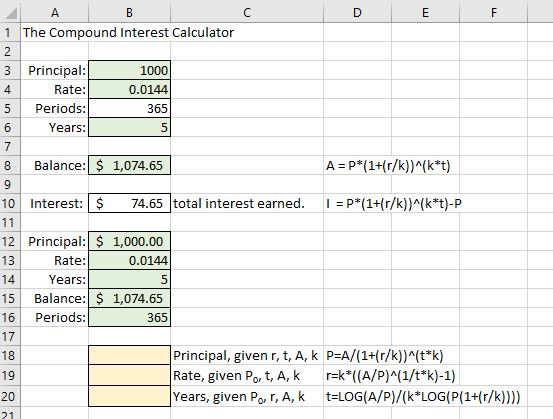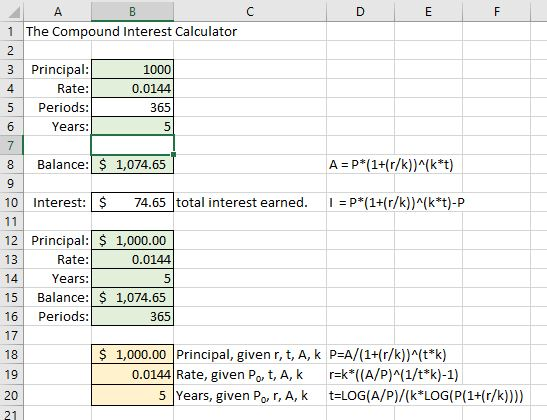## Compound Interest Calculator: Certificates of Deposit

### Learning Outcomes

• Calculate interest compounded over time
• Analyze and compare interest in real-world applications

Certificates of Deposit (CDs) are long-term savings instruments offered by banks. They come in increments such as one, three, five, or 10 years. The idea is that once you deposit your money, you’ll leave it untouched for the duration of the CD term. Banks are usually willing to pay a higher interest in exchange for being assured of using your money longer. But CDs come with a hefty penalty for early withdrawal, so you’ll want to be sure you have the cash to allocate to this type of savings.

You can find easily find annual percentage yields for CDs advertised on banking websites. See the sample below.

Notice that the annual percentage yield is advertised rather than the rate. The APY will always be slightly larger than the rate due to compounding. That is, the interest on your deposit will be calculated at fixed periods throughout the year and reinvested. Recall the formula from earlier in the text.

### Compound Interest

$P_{N}=P_{0}\left(1+\frac{r}{k}\right)^{Nk}$

• PN is the balance in the account after N years.
• P0 is the starting balance of the account (also called initial deposit, or principal)
• r is the annual interest rate in decimal form
• k is the number of compounding periods in one year

Use the formula for compound interest to find the APY for a $1000 5-year CD with a rate of 1.44% compounded daily. Converting Interest Rate to APY We can generalize a formula for converting interest rate to APY: $\text{APY }=1\left(1+\frac{r}{k}\right)^{k}-1$ Banks will usually advertise just the APY since it is a more attractive number. You can find the rate by looking deeper into the details of the CD. Since CDs are designed for you to leave your money in savings for the entire term, they provide excellent examples for exploring compound interest. ### Spreadsheet Hands-On: Create a Compound Interest Calculator ### Step 1: Create the calculator The good news is that we’ve done much of the work to create our calculators already. Since our formulas involve many of the same variables, we can use copy and paste to create the next spreadsheet more quickly. 1. Make a copy of the Simple Interest spreadsheet by right-clicking on the tab containing its name at the bottom of the window, choose Move or Copy, and click the box to Create a copy. Choose (move to end) to place it at the end. Rename your new sheet “Compound Interest” by double-clicking its tab name and typing over it. 2. Using the cells listed in the image below, change the formula in cell B8 to use the compound interest formula by typing into cell B8: =B3*(1+(B4/B5))^(B5*B6).Note that the template formula to the right of that cell has been changed to compound interest as well.3. We’ll need to change the formula in the Interest section, too, from simple interest to compound interest. Since compound interest is reinvested over the life of the CD, the interest accumulated is not constant from year to year. We will only need one interest line. Delete lines 11 and 12 by selecting them, right clicking, then choosing delete. Change the Interest description in C10 to “total interest earned” 4. The response in cell B10 will be the difference between balance and principal : =B8-B3. When you are done, it should look like the image below.### example There are often several good ways to represent a general formula. For instance, the difference between the CD balance at the end of term and the initial deposit is given above simply as the difference between cell B8 and B3. And the formula to the right of cell B12 for total interest earned is also a simple difference. Use algebra to simplify that formula to a general formula for total interest earned. We can also include cells to make speculations about compound interest situations. If we want to ask questions about rate or time given the other variables, we’ll need to rearrange the formula. See the Examples below for the result and step-by-step solutions. ### Recall the power rule for logarithms $\log (M^{n})=n\log M$ This enables you to take the log on both sides to bring the power down. Then, just divide away everything but the variable you wish to isolate. Recall, the formula for compound interest. The balance in the account after $N$ years depends on the principle, rate, compounding periods, and time invested. $P_{N}=P_{0}\left(1+\frac{r}{k}\right)^{Nk}$ ### Example Solve the formula $P_{N}=P_{0}\left(1+\frac{r}{k}\right)^{Nk}$ for $P_{0}$ $P_{0}=\dfrac{P_{N}}{\left(1+\frac{r}{k}\right)^{Nk}}$ ### Example Solve the formula $P_{N}=P_{0}\left(1+\frac{r}{k}\right)^{Nk}$ for $N$ $N=\dfrac{\log\left[\dfrac{P_{N}}{P_{0}}\right]}{k\log\left[\left(1+\frac{r}{k}\right)\right]}$ ### Example Solve the formula $P_{N}=P_{0}\left(1+\frac{r}{k}\right)^{Nk}$ for $r$ $r=k\left[\left(\dfrac{P_{N}}{P_{0}}\right)^{\left(\frac{1}{Nk}\right)}-1\right]$ Now we have the formulas to input for the second portion of the calculator. 1. First, we’ll need to make a little room to include the Periods, since our formulas depend on the number of compounding periods. To add a row, right click on the shaded “16” in row 16 of the sheet. A menu should appear in which you can click “insert” to insert a new line below line 15. Label this line Periods. 2. We’ll need to populate these cells with values that will help us test our formulas. Use the known information from our CD example (see the image below). 3. Also note the new formulas adjacent to B18 – B20. Change yours to match these, which we solved for in the examples above. Delete the simple interest formulas from cell B18, B19, and B20.4. Into cell B18, type =B15/(1+(B13/B16))^(B14*B16) 5. Into cell B19, type =B16*((B15/B12)^(1/(B14*B16))-1) 6. Into cell B20, type =LOG(B15/B12)/(B16*LOG(1+(B13/B16))) 7. The yellow cells should have populated automatically with$1000 for Principal, 0.0144 for Rate, and 5 for Years. If they didn’t, you may have made an error typing in the formula. Check carefully that you have all the parentheses! Getting the formulas into a spreadsheet once may be tedious work, but it is a time-saver in the long run if you use the sheet repeatedly.
8. We won’t need the remaining cells for compound interest. You can delete those lines entirely by clicking on the first line number, dragging down to include them all, right-clicking, and choosing delete.The Compound Interest Calculator is complete!### Step 2: Use the calculator

Use your calculator to investigate the situations that follow.

### example

Suppose you deposit $7,395 into a 3 year CD paying 0.9% interest, compounded monthly. (a) How much money will you have in your account when the CD matures? (b) How much total interest will you have earned? (c) If, on the day of maturity, you reinvest the account balance into a 5 year CD paying 1.29% interest compounded daily, how much money will you have in the account when the second CD matures? (d) How much will your initial investment of of$7,395 have grown over the 8 years it was invested?

### Example

You have $20,000 from an inheritance You would like to invest at least some of it in a CD. (a) You find a CD paying 1.39%, compounded daily for 3 years. What amount would you need to deposit if you want the account balance to be$10,000

(b) What rate would you need to obtain to grow the entire $20,000 to$25,000 over 10 years? You can assume daily compounding.

(c) If you’d like to spend $5,000 now but want the remaining$15,000 to grow back to \$20,000 eventually, what maturity term would you need for a CD paying 1.89% compounded daily?